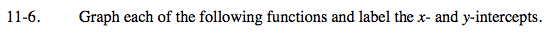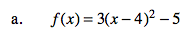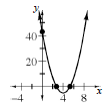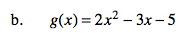Home > A2C > Chapter 11 > Lesson 11.1.1 > Problem11-6

11-6.
1. Graph each of the following functions and label the x− and y-intercepts. Homework Help ✎

1. f(x) = 3(x − 4)2 − 5

2. g(x) = 2x2− 3x − 5Complete the square to changethe function to graphing form.

$g(x) = 2\left(x - \frac{3}{4}\right)^2 - \frac{89}{16}$